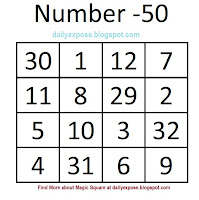### Magic Square (Maths Square)A magic square is a square containing distinct integers such that the numbers in all rows, all columns, and both diagonals sum to the same constant. There are 3X3 squares 4X4, 5X5, ....

Today we will look about 4X4 magic square. I have created a magic square with constant 50.Lets analyse the magic of this square.

If you add the numbers column wise or row wise you will get number 50.

If you add the numbers (30+8+3+9 = 50) diagonally or add the numbers in the four corner boxes (30+7+4+9 = 50) you will get number 50 as total.

Take any 4 adjacent boxes that make a square (2X2) and add the numbers within them and you will amazed to see, that also totals to number 50.

If you add the four corner numbers within 3X3 boxes that make a square and you will find that also totals to the number 50.
If you do the calculations in all possible ways that are mentioned above, (20 ways) you will end up with the total of 50. This is the beauty of this magic square.

So can you create a magic square like this with Number 65 (when you add the numbers in all 20 ways, you have to get Number 65). How to do that? Here is the secret to become maths magician.